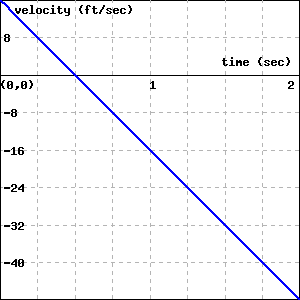A student with a third floor dormitory window 32 feet off the ground tosses a water balloon straight up in the air with an initial velocity of 16 feet per second. It turns out that the instantaneous velocity of the water balloon is given by the velocity function $v(t) = -32t+16$, where $v$ is measured in feet per second and $t$ is measured in seconds.

Let $s(t)$ represent the height of the water balloon above the ground at time $t$, and note that $s$ is an antiderivative of $v$. That is, $v$ is the derivative of $s$: $s'(t) = v(t)$. Find a formula for $s(t)$ that satisfies the initial condition that the balloon is tossed from $32$ feet above ground. In other words, make your formula for $s$ satisfy $s(0) = 32$.
$s(t) =$

At what time does the water balloon reach its maximum height?
$t =$

At what time does the water balloon land?
$t =$

Compute the three differences:
$s\left(\frac{1}{2}\right)-s(0) =$
$s(2)-s\left(\frac{1}{2}\right) =$
$s(2)-s(0) =$
What do these differences represent?

What is the total vertical distance traveled by the water balloon from the time it is tossed until the time it lands?
Total vertical distance =

The graph of the velocity function $y=v(t)= -32t+16$ on the interval $[0,2]$ is shown below.What is the total net signed area bounded by $y = v(t)$ and the $t$-axis on $[0,2]$? You can find the answer to this question in two ways: by using your work above, or by using a familiar geometric formula to compute areas of certain relevant regions.
Total net signed area =

In order to get credit for this problem all answers must be correct.